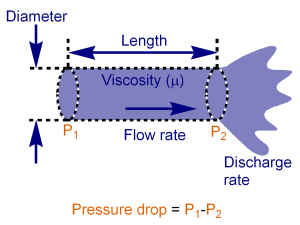# Alicat's principle of mass flow measurement

Alicat mass flow instruments operate on the principle of differential pressure-based laminar flow measurement. Our flow meter and flow controllers are multi-parameter (multivariate) instruments that also display the pressure and temperature data that we use to determine both volumetric and mass flow rates.

## Poiseuille’s equation for laminar flow measurement

One methodology for flow measurement in an internally compensated laminar (ICL) unit is based on the physics of the Poiseuille Equation. First, an internal restriction is created. This restriction is known as a Laminar Flow Element (LFE). The LFE forces the gas molecules to move in parallel paths along the length of the passage, nearly eliminating flow turbulence. (Read about laminar flow here.). The differential pressure drop is measured within the laminar region.The Poiseuille Equation quantifies the relationship between pressure drop and laminar volumetric flow rate as:

Q = (P1-P2)πr4/8ηL

Where:

Q = Volumetric flow rate
P1 = Static pressure at the inlet
P2 = Static pressure at the outlet
r = Hydraulic radius of the restriction
η = Absolute viscosity of the fluid
L = Length of the restriction

Since π, r and L are constant for a given LFE, the equation can be rewritten as:

Q = K(ΔP)/η

In this equation, K is a constant factor determined by the geometry of the restriction. It shows the linear relationship between volumetric flow rate (Q), differential pressure (ΔP) and absolute viscosity (η) in a simpler form.

Changes in gas temperature affect the absolute viscosity of the gas. This requires a temperature measurement to determine the value of η. For most differential pressure-based devices, this is done by manually referencing charts that indicate the viscosity properties of the gas at given temperatures. In an Alicat, this reference is performed continuously through the use of a discrete temperature sensor and a microprocessor.

## Mass flow measurement

At this point, only the volumetric flow rate has been determined. For a laminar flow device to address the range limitations of thermal flow instruments, additional measurements must be taken to determine the actual mass flow rate of the gas. The relationship between volumetric flow and mass flow is:

Mass = Volume * Density Correction Factor

Ideal gas laws show us that the density of a gas is affected by its temperature and absolute pressure. Using ideal gas laws, the effect of temperature on density (at constant pressure) is:

ρas = Ts/Ta

Where:

ρa = Density at flow conditions
Ta = Absolute temperature (°K) at flow conditions in Kelvin
ρs = Density at standard conditions (STP)
Ts = Absolute temperature (°K) at standard conditions (STP) in Kelvin
(°K = °C +273.15)

Similarly, the effect of absolute pressure on density (at constant temperature) is:

ρas = Pa/Ps

Where:

ρa = Density at flow conditions
Pa = Absolute pressure at flow conditions
ρs = Density at standard conditions (STP)
Ps = Absolute pressure at standard conditions (STP)

Therefore, in order to determine the mass flow rate (M), two correction factors must be applied to the volumetric flow rate (Q): the effects of temperature and absolute pressure on density. Together, the conversion to mass flow can be written as:

M = Q(Ts/Ta)(Pa/Ps)

In an Alicat mass flow meter, a discrete absolute pressure sensor is placed in the laminar region of the flow stream. This information is sent to the microprocessor and is combined with the data from the discrete absolute temperature sensor to make the appropriate calculations to determine mass flow.

## Standard temperature and pressure (STP)

Performing the mass flow calculations requires reference to a set of standard temperature and pressure conditions (STP) as indicated by the variables Ts and Ps. STP is usually defined at sea level conditions, but no single standard exists for this convention. Examples of common STP reference conditions include:

0 °C and 1013 mbar
25 °C and 14.696 psia
0 °C and 760 torr or mmHg

It is relevant to note that although the typical units for mass are expressed in grams or kilograms per unit of time (often called “true mass flow”), it has become standard that the mass flow rate is expressed as a standardized volumetric flow rate. Examples include slm/slpm (standard liters per minute), sccm (standard cubic centimeters per minute) and scfh (standard cubic feet per hour). By knowing the STP setting of the device and the density of a particular gas at that STP, it is possible to determine the flow rate in grams per minute, kilograms per hour, etc., as the following example shows.

Given:

Gas = Helium
M = 250 SCCM
STP = 25 °C and 14.696 PSIA
Gas Density = 0.166 Grams per Liter

True Mass Flow = M * Gas Density at STP
True Mass Flow = (250 SCCM)(1 liter per 1000 CC)(0.1636 grams per liter)
True Mass Flow = 0.0409 Grams per Minute of Helium

Alicat mass flow meters and mass flow controllers made since spring 2016 can display mass flow rates as true mass flow rates. Simply select the desired unit, and your Alicat will perform all the calculations.